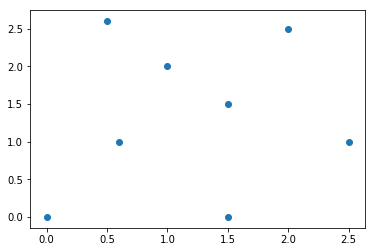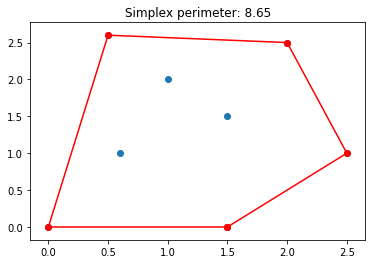# The Convex Hull Problem¶

Dec. 18, 2018

Consider the following simple coding exercise:

Given a set of points in $\mathbb{R}^2$ with nonnegative coordinates, find the perimeter of the convex hull formed by these points.

We use Python math and matplotlib to solve this problem.

In :
import math
import matplotlib.pyplot as plt


There are many algorithms for finding the convex hull. A famous algorithm is called the Graham scan. Rather than using that, I came up with another more intuitive method, which goes as follows:

1. Pick the first basis point whose polar representation has the smallest angle.
2. Rotate all points with respect to their center of mass by angle $\theta$, and find the second point with the smallest polar angle.
3. The process is repeated until all points are rotated to their original position with a suitably chosen angle $\theta$.
4. The results (minus repeats) are a set of points (in counter-clockwise order) that define the convex hull of all points.

This is a great coding exercise! First, let's define two helper functions. The function norm calculates the length of vector. The function argmin outputs the minimum index given a list of real numbers.

In :
# length of vector (x, y)'
def norm(y,x):
return (y**2+x**2)**.5

# find index of minimum item in a list
def argmin(iterable):
return min(enumerate(iterable), key=lambda x: x)


The conversions among polar and Cartesian coordinates can be achieved by the functions to_polar and to_cart. Furthermore, the function get_basis finds the point with the minimum polar angle.

In :
# convert Cartesian to polar
def to_polar(X):
X_polar = []
for x in X:
length = norm(x,x)
angle = math.atan2(x,x)
X_polar.append([length,angle])
return X_polar

# convert polar to Cartesian
def to_cart(X):
X_cart = []
for [r, theta] in X:
xx = r*math.cos(theta)
yy = r*math.sin(theta)
X_cart.append([xx, yy])
return X_cart

# obtain a point that touch the rays from origin
def get_basis(X):
Xp = to_polar(X)
theta = [Xp[i] for i in range(len(X))]
return argmin(theta)


To rotate the simplex with respect to their center of mass, first standardize all points so that they are centered at the origin. Next, find the minimum angle between two points and use that as the step size for the rotation. After rotation, we return all points back to their original position. The following helper functions achieve this goal.

In :
# center the points at origin
def standardize(X):
Xs = []
x = [X[i] for i in range(len(X))]
y = [X[i] for i in range(len(X))]
xbar = sum(x)/len(x)
ybar = sum(y)/len(y)
for i in range(len(X)):
Xs.append([X[i]-xbar, X[i]-ybar])
return Xs, xbar, ybar

# un-center the points
def recover(Xs, xbar, ybar):
X = []
for i in range(len(Xs)):
X.append([Xs[i]+xbar, Xs[i]+ybar])
return X

# obtain the angle of rotation
def min_angle(X):
Xs, _, _ = standardize(X)
angles = sorted([to_polar(Xs)[i] for i in range(len(Xs))])
angle = min([angles[i]-angles[i-1] for i in range(1, len(angles)) if angles[i]-angles[i-1]!=0])
return angle

# rotate all points clockwise by "angle"
def rotate(X, angle):
Xs, xbar, ybar = standardize(X)
Xp = to_polar(Xs)
for i in range(len(Xp)):
Xp[i] += angle
Xc = to_cart(Xp)
X = recover(Xc, xbar, ybar)
return X


Finally we are ready to initialize our method.

In :
class simplex():
"""
X = [[x1,y1], [x2,y2],...]
"""
def __init__(self, X):
self.X = X  # set of points
self.basis = []  # storage for basis points

# find convex hull
def fill_basis(self):
self.basis = []
min_theta = min_angle(self.X)
basis_set = []
for i in range(math.floor(math.pi*2/min_theta)):
Xr = rotate(self.X, min_theta*i)
new_basis = get_basis(Xr)
if len(basis_set) == 0:
basis_set.append(new_basis)
else:
if new_basis != basis_set[-1]:
basis_set.append(new_basis)
for i in basis_set:
self.basis.append(self.X[i])
return self.basis

# find perimeter of convex hull
def area(self):
if len(self.basis)!=0:
a = 0
xb = [self.basis[i] for i in range(len(self.basis))]
yb = [self.basis[i] for i in range(len(self.basis))]
for i in range(len(xb)-1):
a += norm(yb[i]-yb[i+1], xb[i]-xb[i+1])
a += norm(yb[len(xb)-1]-yb, xb[len(xb)-1]-xb)
return a
else: return 0

def plot(self):
x = [self.X[i] for i in range(len(self.X))]
y = [self.X[i] for i in range(len(self.X))]
plt.scatter(x, y)

if len(self.basis) != 0:
xb = [self.basis[i] for i in range(len(self.basis))]
yb = [self.basis[i] for i in range(len(self.basis))]
plt.scatter(xb, yb, c = 'r')
for i in range(len(xb)-1):
plt.plot([xb[i], xb[i+1]], [yb[i], yb[i+1]], c='r')
plt.plot([xb[len(xb)-1], xb], [yb[len(xb)-1], yb], c='r')
plt.title('Simplex perimeter: {:.2f}'.format(self.area()))


For demonstration, let's define a set of points.

In :
X=[[1.5,0],[1,2],[0.6,1],[0.5,2.6],[2,2.5],[1.5,1.5],[0,0],[2.5,1]]
S = simplex(X)
S.plot()In :
S = simplex(X)
print(S.fill_basis())

[[1.5, 0], [0, 0], [0.5, 2.6], [2, 2.5], [2.5, 1], [1.5, 0]]

In :
S.plot()